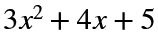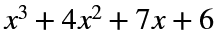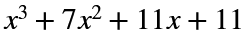# Problem 44274. Calculate the sum of two polynomials

Calculate the sum of two polynomials if they are written in notation with their coefficients.
example:
() + () =a=[3 4 5];
b=[1 4 7 6];
output =[1 7 11 11];

### Solution Stats

53.99% Correct | 46.01% Incorrect
Last Solution submitted on Dec 01, 2023

### Community Treasure Hunt

Find the treasures in MATLAB Central and discover how the community can help you!

Start Hunting!